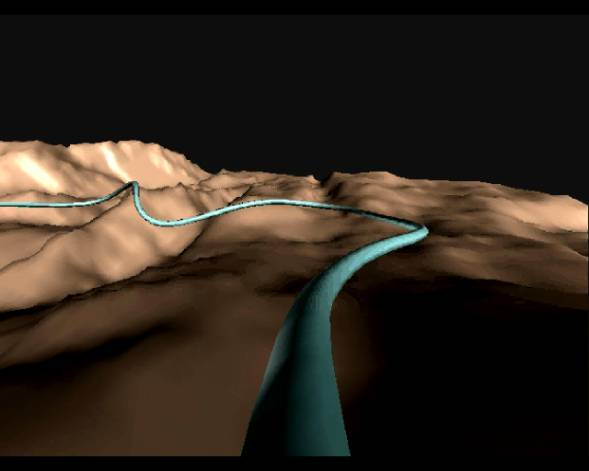The Orthogonal Direction Change Chain Code

Chain-code techniques are widely used because they preserve information and allow considerable data reduction, chain codes are the standard input format for numerous shape analysis algorithms. Using the orthogonal direction change chain code it is possible to represent three-dimensional (3D) curves. Any 3D continuous curve can be digitalized and represented as a 3D discrete curve. This 3D discrete curve is composed of orthogonal constant straight-line segments. Thus, the chain elements represent the orthogonal direction changes of the constant straight-line segments of the discrete curve. The proposed chain code only considers relative direction changes, which allows us to have a unique curve descriptor invariant under translation and rotation. Also, this curve descriptor may be starting point normalized for open and closed curves and invariant under mirroring transformation.

Examples:This curve was represented using the orthogonal direction change chain code.A polygonal path of a Hilbert sequence represented by means of the orthogonal direction change chain code.A polygonal path of a Hilbert sequence represented by means of the orthogonal direction change chain code.

References:

• Bribiesca E., A Chain Code for Representing 3D Curves, Pattern Recognition, Vol. 33, No. 5, pp. 755-765 (2000).
• Bribiesca E. and Velarde C., A Formal Language Approach for a 3D Curve Representation, Computers & Mathematics with Applications, Vol. 42, No.12, pp. 1571-1584 (2001).
• Bribiesca E., Scanning-Curves Representation for the Coverage of Surfaces Using Chain Coding, Computers & Graphics, Vol. 27, pp. 123-132  (2003).
• Bribiesca E., 3D-Curve Representation by Means of a Binary Chain Code, Mathematical and Computer Modelling, Vol. 40 (3-4), pp. 285-295, (Aug 2004).